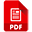# That Blue Square Thing

Syllabus content:Unit 3 character content - just character encoding
Note: this syllabus content is a slightly amended version of the one published freely on the web by AQA. I have made very minor adjustments to remove some content less suitable for students to use and it is presented here simply to allow the children I teach to download a usable copy of the syllabus content. It is copyright AQA and reproduced here simply to make access easier for students. No attempt to claim copyright is being made, although I could have copied the text into my own interpretation...

## AQA Computer Science GCSE

This page is up to date for the new AQA 8525 syllabus for the 2022 exam.

### Data Representation - Representing Text and Characters

Everything stored inside a computer has to ened up being represented using just binary digits. That means everything has to become a series of numbers. That includes writing (just like this paragraph that you just read...).

To do this, each character on a keyboard needs to have a number associated with it. That means that each letter, punctuation mark, symbol and digit needs to be able to be represented using a number. This includes spaces and some "non-printing characters" - for example, the "character" which represents a new line.

This was first done using ASCII code - a series of 128 character codes to represent characters. But 128 characters isn't enough to deal with modern computers, so the system was extended using Unicode. You need to know how both work and why they work the way they do.ASCII code - slides from classUnicode - slides from classASCII and Unicode - double page spreadThe 128 character ASCII code table - this uses 7 bit binary

Note that ASCII uses 7-bit binary to encode each character. So, to calculate the data required to hold a word, you multiply the number of characters by 7.

So, the word "tomato" has 6 characters, each encoded using 7 bits. It needs 6 x 7 bits = 42 bits to save the word.

This becomes important when you start to use data compression to reduce file sizes.

ASCII is an example of a computing standard.What is a standard?

#### Working with Characters and Strings

When you manipulate string variables in programming languages, the ASCII (or Unicode) values for each character are actually how the programming language deals with the variables. The slides below will help explain how this works.Comparing String Variables

#### Converting Characters to Codes:

You can convert a character to its ASCII code representation in most programming languages. This can be helpful sometimes and is something you need to know for exams.

Some pseudocode to do this is shown below:

theName <- "Doris Budge"

FOR i <- 0 TO LEN(theName) - 1 #LEN gives the length of the string
theChar <- CHAR_TO_CODE(theName[i])
OUTPUT theChar
ENDFOR

This code iterates over the string letter by letter using the FOR loop. CHAR_TO_CODE then converts the next character to its ASCII code number and prints that out (so, D is 68, o is 111 etc...). The space in the name will be converted to 32 - the ASCII code value for a space character.

Some Python to do the same thing is shown below:

theName = "Doris Budge"

for i in range(0, len(theName)):
theChar = ord(theName[i])
print(theChar)

ord() is the Python equivalent of CHAR_TO_CODE. Perhaps the only time that Pseudocode is easier to use than Python!

A slightly different way of iterating over the string in Python is shown below. This is easier to use when you have to use a FOR loop.

theName = "Doris Budge"

for letter in theName:
theChar = ord(letter)
print(theChar)

This method means you don't have to keep track of the value of i.

#### Converting Codes to Characters:

You can also convert from a character code to a character using CODE_TO_CHAR:

theCode <- USERINPUT

theChar <- CODE_TO_CHAR(theCode)
OUTPUT theChar

This code uses USERINPUT to allow you to enter a character code. When you enter a number in Python it gets stored as a string, so when using Python we need to make sure we convert to an integer first.

theCode = int(input("Enter a character code: "))

theChar = chr(theCode)
print(theChar)

This time Python uses the built in function chr() to convert from an integer to a character.

You may need to use Pseudocode to convert to or from a string (or, more likely, to be able to work out what the exam board's pseudocode is doing). This is easy enough:
• STRING_TO_INT(aString) - converts from a string to an integer
• INT_TO_STRING(anInteger) - the opposite
• STRING_TO_REAL(aString) - converts from a string to a real number (a decimal number, e.g. 3.142)
• REAL_TO_STRING(aRealNumber) - the opposite

#### More on Strings

There is more on how strings work on the sub-page for this topic.